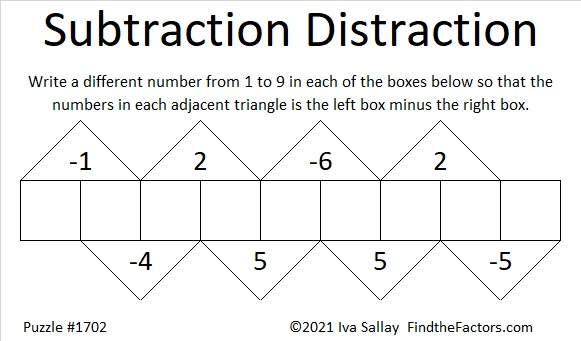# 1702 A Puzzle Idea from @mathequalslove Tweaked into a Subtraction Puzzle That Directs You to a Post from NebusResearch

Contents

### Today’s Puzzle:

Joseph Nebus is nearly finished with all the posts in his Little 2021 Mathematics A to Z series. Every year he requests that his readers give him mathematical subjects to write about. At my suggestion, he recently wrote about subtraction, and how it is a subject that isn’t always as elementary as you might expect.  With a touch of humor, we learn that subtraction opens up whole new topics in mathematics.

I wanted to make a puzzle to commemorate his post. I gave it some thought and remembered a tweet from Sarah Carter @mathequalslove:

That puzzle originated from The Little Giant Encylopedia of Puzzles by the Diagram Group. I wondered how the puzzle would work if it were a subtraction puzzle instead of an addition puzzle, and here’s how I tweaked it:There is only one solution. I hope you will try to find it! If you would like a hint, I’ll share one at the end of this post.

### Factors of 1702:

• 1702 is a composite number.
• Prime factorization: 1702 = 2 × 23 × 37.
• 1702 has no exponents greater than 1 in its prime factorization, so √1702 cannot be simplified.
• The exponents in the prime factorization are 1, 1, and 1. Adding one to each exponent and multiplying we get (1 + 1)(1 + 1)(1 + 1) = 2 × 2 × 2 = 8. Therefore 1702 has exactly 8 factors.
• The factors of 1702 are outlined with their factor pair partners in the graphic below.### More About the Number 1702:

1702 is the hypotenuse of a Pythagorean triple:
552-1610-1702, which is (12-35-37) times 46.

1702² = 2896804, and
2197² = 4826809.
Do you notice what OEIS.org noticed about those two square numbers?

### Puzzle Hint:

Here’s how I solved the puzzle: I let the rightmost box be x. Then using the values in the adjacent triangles and working from right to left, I wrote the values of the other boxes in terms of x.x – 5 went in the box that is second to the right,
x – 5 + 2 = x – 3 went in the next box,
x – 3 + 5 = x + 2,
x + 2 – 6 = x – 4,
x – 4 + 5 = x + 1, and so on until I had assigned a value in terms of x for every box.

Think about it, and this hint should be enough for you to figure out where the numbers from 1 to 9 need to go.

## One thought on “1702 A Puzzle Idea from @mathequalslove Tweaked into a Subtraction Puzzle That Directs You to a Post from NebusResearch”

This site uses Akismet to reduce spam. Learn how your comment data is processed.